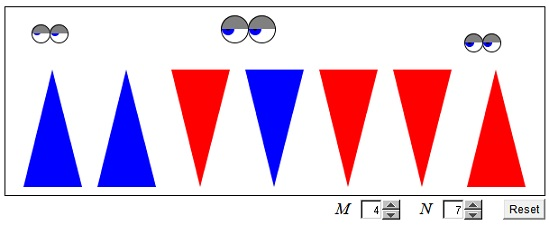# Flipping Items Simultaneously

The applet displays several (N) triangles, all pointing upwards initially. On any move, you can turn over any M of them. (You do that by clicking on M triangles in turn.) The question is, Is it possible to have all N triangles point downwards?

### If you are reading this, your browser is not set to run Java applets. Try IE11 or Safari and declare the site https:///www.cut-the-knot.org as trusted in the Java setup.What if applet does not run?

The original puzzle [Mathematical Circles, p. 132] stated for N = 7 and M = 4 has negative solution. It is easily seen that, in this case, the number of inverted triangles is always even and, therefore, can't be 7. In [Trigg, #22], M = N - 1, and it is shown that the puzzle is solvable for N even and is not solvable for N odd.

The solution begs for a generalization: what can be said about other pairs of M and N?

## References

1. D. Fomin, S. Genkin, I. Itenberg, Mathematical Circles (Russian Experience), AMS, 1996
2. C. W. Trigg, Mathematical Quickies, Dover, 1985, #22.Quite obviously, if the problem is solvable for a given pair M, N then it is solvable for the pair qN, qM, with q a positive integer. For a long time I thought that the converse is also true, i.e. that the problems for (N, M) and the (reduced) pair M/gcd(M, N), N/gcd(M, N) are quivalent. I was advised by the Zbarsky family that they are not. (For example, for N = 3 and M = 2 the problem has no solution. However, it is solvable for N = 6 andd M = 4 in just three steps.)

For M and N are mutually prime, the puzzle is solvable wherever M is odd, and unsolvable otherwise. Why?

It is easy to see that when N is odd and M is even, the puzzle is unsolvable. Indeed, assign number ±1 to each of the triangles depending whether it points up or down. Note the product Π of all the assigned numbers. When all triangles point upwards, Π = 1. When all point downwards, Π = -1. If M is even then flipping M triangles does not affect Π, and therefore, in this case, it is impossible to flip all the triangles.

If M = N - 1, there are C(N, N-1) = N combinations of N-1 elements out of N. Each of N elements enters N-1 of the combinations. Carrying out the flips corresponding to all N combinations, will turn each of the N triangles N-1 times, which is an odd number, and therefore leave it in a position different from the one it was originally in, i.e., upside down.

It remains to be shown that when gcd(M, N) = 1 and M odd the puzzle is always solvable. One solution can be found at my blog.

It so happens that the case of an odd N is much easier than the case where N is even. For odd N, the problem can always be solved in 3 steps. When N is even, one needs at least 4 moves.